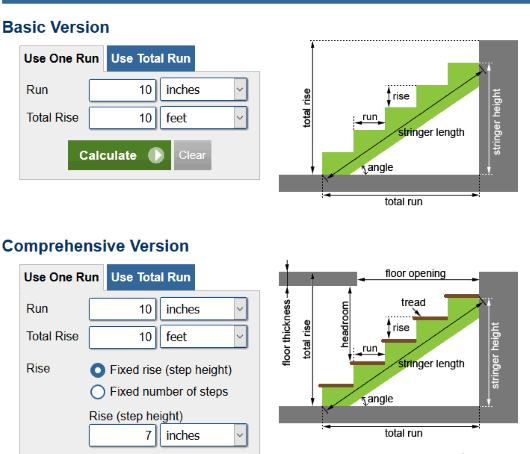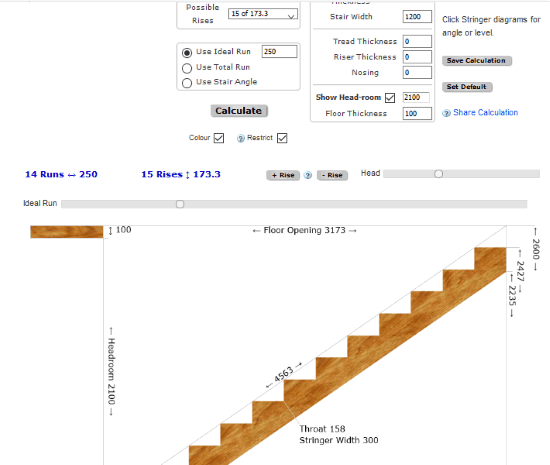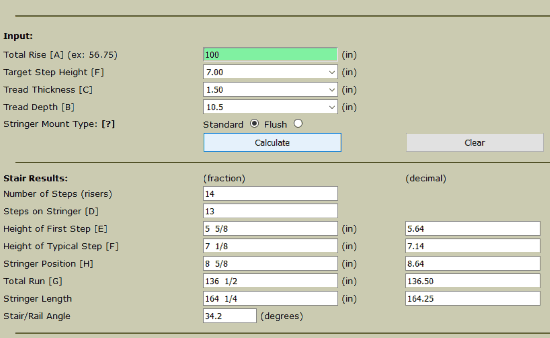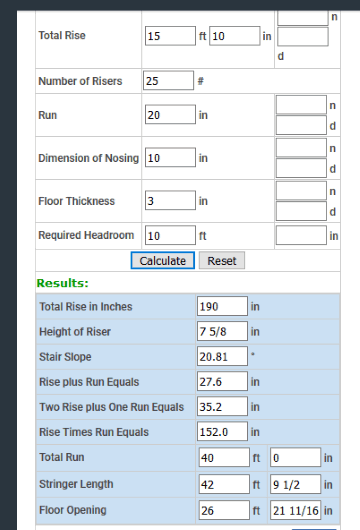Editor Ratings:
User Ratings:
[Total: 3 Average: 4]

Here is a list of 4 free online stair calculators. Stair calculation is used for calculating different parameters involved in stairs construction. Using these sites, you will be able to calculate stair angle, stair rise and run, height of first step, stringer length, stringer height, total run, and more.

Some of these stair calculator websites also provide sample graph where you can see total run, stringer length, stringer height, etc., to understand about stair calculation. You can enter input details and then get the output parameters for stair calculation.

Let’s check these free online stair calculators.

## Calculator.netCalculator.net brings a very useful Stair Calculator tool. Using that tool, you can use basic or comprehensive version and then perform stair calculation with one run and total rise or total run and total rise. It also provides a sample diagram to understand about various parameters for stairs calculation.

When you enter the values and use Calculate button, you will see the result. The result comes for different steps (like 12 steps, 13 steps, etc.). You can check number of rise, number of run, rise (in inches or cm), run (in feet or cm), stair angle, stringer height, and length.

## Blocklayer.comBlocklayer.com brings important options for stair calculation. You can set:

• Total rise.
• Stringer width or concrete thickness (if you want to build concrete stairs).
• Ideal rise (using a value with the help of drop down menu).
• Possible rise.
• Show/hide head-room with a custom value.
• Use total run/ideal run/stair angle.

After adding these details, you can use Calculate button. The result shows different graphs. In the graph, you can check stringer width, base length, headroom size, floor opening, etc. You can also show running string points or view those points in the graph only.

## Mycarpentry.comMycarpentry.com website also brings a good stair calculator. The interface is very basic but result comes as expected. You can enter details like total stair rise, tread thickness, tread depth, stringer mount type (standard or flush), target step height, and then use the Calculate button.

The results will show details for number steps (or risers), steps on stringer, height of first step, typical step, total run, stair angle, etc. It also provides stair calculator diagram based on the input values and results, which is a very good feature of this stair calculator website.

## Spikevm.comSpikevm.com website has a very simple interface and stair calculator. Just enter your stair calculator details like total rise, number of rises, dimension of nosing, required headroom, run, floor thickness, etc., and then you can generate the result.

The output will show total rise in inches, two rise plus one run equals, total run, floor opening, stair slope angle, stringer length, etc. This is all this feature does. It doesn’t show any graph for output but available options are good enough for stair calculation.

### The Conclusion:

These are the good online stair calculators that you may try. All are good to perform the basic calculation. But, for me, the first website is good. It comes with basic and comprehensive versions where you can perform stair calculation with one run and total rise or total run and total rise.

 Editor Ratings: User Ratings:[Total: 3 Average: 4] Tags: calculator